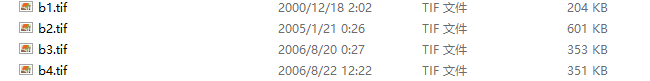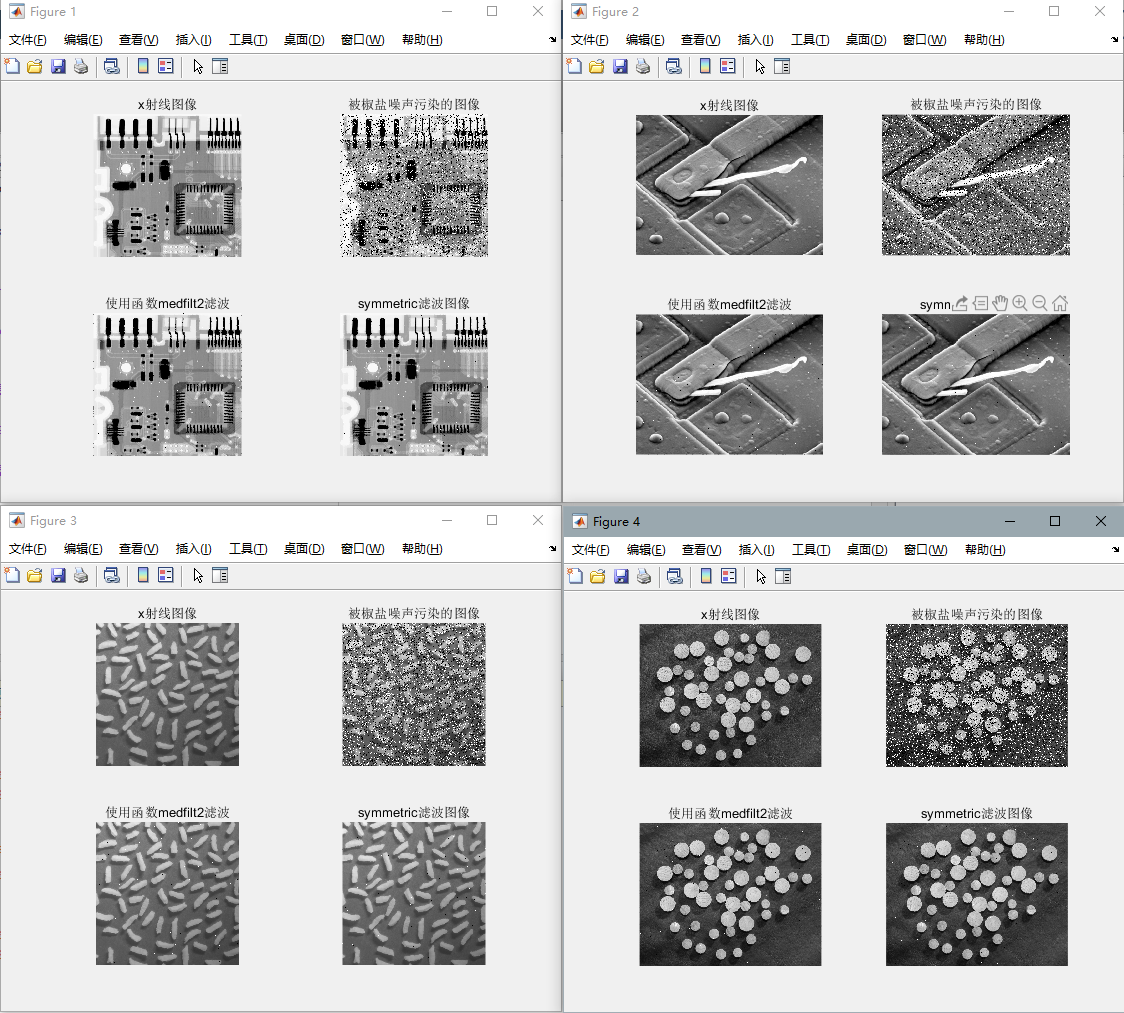• matlab循环保存图片并命名
千次阅读
2019-09-21 15:48:47

for i = startFrame:endFrame
frames = reshape(Y(:,i), Height, Width);
imwrite(frames,['D:/',num2str(i),'.jpg']);  #命名并保存，要注意i是数字要转换成字符串
end

更多相关内容
• for循环中，保存图片，并命名不同的名字 fileName = ['特征值1_幅值标准差/',titleName,'.png']; print(fileName,figure1,'-dpng','-r600'); 特征值1_幅值标准差/是要保存的文件夹名字，titleName是图片要保存的...

for循环中，保存图片，并命名不同的名字

fileName = ['特征值1_幅值标准差/',titleName,'.png'];
print(fileName,figure1,'-dpng','-r600');

特征值1_幅值标准差/是要保存的文件夹名字，titleName是图片要保存的名字

展开全文该楼层疑似违规已被系统折叠 隐藏此楼查看此楼

[m,n]=size(rice);

I2=double(rice);    %变换矩阵中的每个元素

for i=1:m

for j=1:n

for k=0:7

f=I2(i,j);

g(i,j)(k)=0;

if (f>=0)&&(f<=2^k)

g(i,j)(k)=0;

elseif(f>=2^k)&&(f<=(2^8-1))

g(i,j)(k)=1;

end

end

end

end

subplot(2,5,1);subimage(rice);title('(1) original image')

subplot(2,5,2);subimage(mat2gray(g(0)));title('(2) 0 bitmap image')

subplot(2,5,3);subimage(mat2gray(g(1)));title('(3) 1 bitmap image')

subplot(2,5,4);subimage(mat2gray(g(2)));title('(4) 2 bitmap image')

subplot(2,5,5);subimage(mat2gray(g(3)));title('(5) 3 bitmap image')

subplot(2,5,6);subimage(mat2gray(g(4)));title('(6) 4 bitmap image')

subplot(2,5,7);subimage(mat2gray(g(5)));title('(7) 5 bitmap image')

subplot(2,5,8);subimage(mat2gray(g(6)));title('(8) 6 bitmap image')

subplot(2,5,9);subimage(mat2gray(g(7)));title('(9) 7 bitmap image')

结果显示??? Error: File: bitmap.m Line: 9 Column: 9

()-indexing must appear last in an index expression.

请问应该怎样修改，非常谢谢！

展开全文• Matlab 数字图像处理 for循环处理多张图片 需求 对文件名b1.tif ; b2.tif ; b3.tif ; b4.tif 进行同一图像处理操作 用for函数循环输出，避免繁琐操作 关键函数 num2str(i) 实现对文件名编号的读入 代码 for i=1...

# Matlab 数字图像处理 for循环处理多张图片

## 需求

1. 对文件名b1.tif ; b2.tif ; b3.tif ; b4.tif 进行同一图像处理操作2. 用for函数循环输出，避免繁琐操作

num2str(i)
实现对文件名编号的读入

## 代码

for i=1:4
i1=imnoise(image,'salt & pepper',0.2)
i2=medfilt2(i1)
i3=medfilt2(i1,'symmetric')
figure,
subplot(221),imshow(['b',num2str(i),'.tif'])
title('x射线图像')
subplot(222),imshow(i1)
title('被椒盐噪声污染的图像')
subplot(223),imshow(i2)
title('使用函数medfilt2滤波')
subplot(224),imshow(i3)
title('symmetric滤波图像')
end


## 输出展开全文• 问题描述：matlab输出图像数据的一个题i=imread('clown.jpg');[x,y]=size(i);for j=1:x,for k=1:y,i(j,k)endend为什么输出ans和i矩阵中相对应的元素有些相同有些不同呢 怎么回事 是for用错了 还是数据格式问题 ...
• 就比如我要用MATLAB绘制图像，其中 C1：C100；C101:C200……这样一组组取数，想要把这些数据绘制成一幅幅图像，每一组数据对应一幅图像。由于总共有一百多组数据没办法一个个分开来读，想用循环的话，该怎么写代码呢...深度学习
• f1 = 1:35; v11(1) = 0; v10(1) = 0;... （循环内容） end figure %因为矩阵从1开始 故需要回减1 plot(f1-1,a11,f1-1,a10); ylabel('纵坐标信息'); xlabel('横坐标信息'); f1为设置的长度。用f1的长...
• function getctimdata(savefilepath) Difo=dir([savefilepath ‘*.tif’]); FNtif={Difo.name}; nn=length(FNtif);... end 在命令窗口，输入 getctimdata(‘文件名\’)两万处理的图片集文件夹替换文件名然后运行
• 本帖最后由 black_angel 于 2015-5-15 14:33 编辑Zhang-Suen的细化算法的matlab程序中，使用了while循环来实现细化，提取骨架。代码如下：clc,clearaddpath('D:\Program files\MATLAB\R2013a\bin\Thinning\Thinning\...
• ## matlab循环生成矩阵

千次阅读 2021-04-21 06:26:41
MATLAB 的矩阵求值的循环语句?clc;clearall;closeall;t0=;a=[12;34]t=t0;t(1,:)=t0'\an=10;fori=2:nt(i,:)=t(i-1,:)'\a;endtmatlab 中的核矩阵for循环问题A(i,:)-A(j,:)表示A矩阵的第i行减去第j行,得到的是一个...
• I have segmented results in a folder. Those need to be read in a for loop and processed further in the loop. I tried reading as below:for i=1:10file_name=dir(strcat('C:\Users\adminp\Desktop\dinosaurs\...
• 这是代码：for i= 1:4;fileID = fopen('testdata.txt', 'at');fprintf(fileID, 'this is answer %d\n',i);fprintf(fileID, '%5.3e\n', v{i});fclose(fileID);end在记事本中回答：this is answer 11.000e+0001.000e+...
• matlab批量读取图片代码PyTorch-ARCNN 这是为ARCNN编写的非官方测试专用脚本。 它加载预训练的权重（.m）并在PyTorch中执行。 绝对没有培训功能。 有关详细信息，请访问论文作者的网站：。 这可以作为其他图像处理...
• Matlab - 如何输出for循环作为一个表，每次迭代和结果显示(Matlab - How to output a for loop as a table with each iteration and result displayed)我想知道如何在Matlab输出for循环，以便最终得到一个表，其中...
• 怎样将MATLAB中的数据输出到excel中使用xlswrite()函数可以将matlab中数据保存到excel中，请看下面例子：>>data=magic(5)%示例数据data=17241815235714164613202210121921...
• close all; clc; ...% 当读取的图片在程序文件夹的子文件夹中是需调用此命令 m = 24; % 10个图片文件 P = []; for k = 1:m  P = imread('image.pgm']);  subplot(4,6,k);  imshow(P);
• 这两天要给导师做实验，因此要写Matlab程序，在做程序的时候，涉及到了循环读入和输出，查找相关资料 代码如下 for i=1:129 Image=['F:\\SYTP\\',num2str(i),'.jpg']; Texture(Image); eval(['save F:\\...
• Matlab循环中如何实现print自动存图片 如：print('-dtiff','-r300','w.tiff'); 此处，本意想以变量w （1 2 3 ...）作为tiff图片的文件名，而此程序序号无法实现增加，新图片将旧的覆盖掉了。请问如何才能实现用...print
• Plot(...)任意的绘图， Text(x, y , ['Monte Carlo Number=' num2str(mc) '/' num2str(NMc)])将这个语句写在循环体的内部。 5 在保存图像的时候用到动态的给文件起名的时候，也可以用这个语句。文件名[‘file’ ...
• 我想用matlab批量读取文件夹内的所有图片，最开始我计划使用dr来获取文件夹下图片的名称然后读取，但是那样会报错没有权限。于是我计划创建一个单独的数组来储存每个文件的路径，代码如下 file1 = dir('images\neg\*...有问必答
• 第二种方式是用copy命令copy成metafile...matlab下的xlabel项，grid，坐标刻度，曲线的粗细，线型等都可以任意编辑。图形的大小可在关闭图片状态下用鼠标任意修改，还可以用鼠标加shift键剪去你不想要的部分。你可以...
• 例子:处理一文件夹下的所有文件，读取每个文件里的数据并利用相应数据画图，保存每个图片。代码:clcclearf_structure=dir('F:Cruise_data_973_II_200806Ts0805');%--put allthe files of pwd in a structurefor i=3:...
• 在每次迭代后暂停循环并显示结果MATLAB(pausing for loop after every iteration and display the results MATLAB)我有以下代码，我在循环中调用函数并在每次迭代后暂停它：[num,txt1,~]=xlsread('test.xlsx',1);...
• savegif 将位于for循环中的figure动画转化为gif文件 用法： savegif必须在for循环中输入savegif函数，默认文件名为‘untitledgif.gif’ savegif（'filename'）必须在for循环中使用，文件名保存为‘filename.gif’. ...
• 最近需要做CNN，需要用到大量的仿真图，但是一个一个保存肯定...saveas(h1, ['D:\AAA资料\Matlab\保存的图\LG叠加态\tu', num2str(A+9), '.tiff']); 但是这样保存出来有白色的边框，还是得用imwrite for A = 1:1
• matlab图像里面的x，y坐标轴的数据输出可以用一个循环，假设x轴数据存储在x中，y轴数据存储在y中，另设两个变量a和b分别存储各张图像的x和y的数据。可以用matlab提供的坐标轴设置函数axis进行设置(对当前坐标轴......

# matlab循环输出图像matlab 订阅